## Saturday, November 30, 2013

### Here Comes Official OpenCV-Python Tutorials

Hi,

First of all, our blog visitor count is about to cross 4 lakhs. Thanks to all for your support !!!

So a small gift on this occasion ...

A couple of months ago, I had told you about a new OpenCV-Python tutorial was under development.

So it is the time to unveil it. You can visit the new official tutorial at OpenCV website.

Remember, it is based on the not-yet-released OpenCV 3.x version. To get it, you have to compile OpenCV from source available in Github. (Because, I always get mail saying some there are some errors in tutorial, it doesn't work. Actually they use OpenCV 2.x version. That is the problem)

It was a great pleasure to work on this project. I thank my mentor, Mr. Alexander Mordvintsev for his help on this project. I also thank many OpenCV developers like Gary Bradsky, Vadim Pisarevsky, Vincent Rabaud etc. for their help.

So friends, please read it, enjoy it, and don't forget to send me your comments, thoughts, feedbacks, bug reports, feature requests etc. I know it is still incomplete. We will together make it complete !!!

Thank you all,

Regards

Abid Rahman K.

## Saturday, July 13, 2013

### Grabcut Algorithm in OpenCV

Hi friends,

Grabcut algorithm is a nice tool for foreground-background extraction with minimal user interface. It is developed by Microsoft research labs.

See one result below:

You start by drawing a rectangle around the foreground image. Algorithm then segments the image. There can be some misclassifications. There you provide some nice touchups specifying this area is background, this area is foreground etc. Again segment the image to get very nice results.

You can find a python sample at OpenCV source at this link. Watch a video demo of the same code below:

For more details of the algorithm and code, please visit my new tutorial repo: https://github.com/abidrahmank/OpenCV2-Python-Tutorials

Currently, no build is available, so please fork the repo, clone it and build it using sphinx. (Or download zip file if you don't have git account and then build it).

Sphinx installation is just one command with easy_install. Please visit here: http://sphinx-doc.org/

Cheers,
Abid K

## Friday, June 7, 2013

### " Towards the end of Journey "

Hi friends,

This is a good news or a bad news.

( Bad News ) Most probably, I won't be posting anymore tutorials on this blog.

( Good News ) Within a couple of months, all the tutorials in this blog + a few extra, will be available as online HTML or a PDF document in OpenCV official documentation site (www.docs.opencv.org).

But this time, I am not alone. @Alexander Mordvintsev, a software engineer from Moscow, will be helping me in this journey.

Work is still in its initial stage. Those who want to get a copy of the tutorials, please visit the github repo : https://github.com/abidrahmank/OpenCV2-Python-Tutorials

Fork it, Clone it, Use it.

All details are there in README file. It is created with Sphinx and it looks more better. You will need sphinx to build it.

Since it is in progress, you may find errors and all other unexpected stuffs. Please inform me if you find anyone. You can comment in my blog, you can mail me at abidrahman2@gmail.com, or you can create an issue in git repo.

I will be posting news about development of the work, stay tuned...

Meanwhile, I would like to hear your opinions, comments, feedbacks,... everything :)

Regards

Abid Rahman K.

# Thresholding

Hi friends,

### Simple Thresholding

Here, the matter is straight forward. If pixel value is greater than a arbitrary value, it is assigned one value (may be white), else it is assigned another value (may be white).
The function used is threshold(). First param is the source image, which should be a grayscale image. Second param is the threshold value which is used to classify the pixel values. Third param is the maxVal which represents the value to be given if pixel value is more than (sometimes less than) the threshold value. OpenCV provides different styles of thresholding and it decided by the fourth parameter of the function. Different types are:
1. cv2.THRESH_BINARY
2. cv2.THRESH_BINARY_INV
3. cv2.THRESH_TRUNC
4. cv2.THRESH_TOZERO
5. cv2.THRESH_TOZERO_INV
Two outputs are obtained. First one is a retval which I will explain later. Second output is our thresholded image.

import cv2
import numpy as np
from matplotlib import pyplot as plt

ret,thresh1 = cv2.threshold(img,127,255,cv2.THRESH_BINARY)
ret,thresh2 = cv2.threshold(img,127,255,cv2.THRESH_BINARY_INV)
ret,thresh3 = cv2.threshold(img,127,255,cv2.THRESH_TRUNC)
ret,thresh4 = cv2.threshold(img,127,255,cv2.THRESH_TOZERO)
ret,thresh5 = cv2.threshold(img,127,255,cv2.THRESH_TOZERO_INV)

thresh = ['img','thresh1','thresh2','thresh3','thresh4','thresh5']

for i in xrange(6):
plt.subplot(2,3,i+1),plt.imshow(eval(thresh[i]),'gray')
plt.title(thresh[i])

plt.show()

Result :

In the previous section, we used a global value as threshold value. But it may not be good in all the conditions where image has different lighting conditions in different areas. In that case, we go for adaptive thresholding. In this, the algorithm calculate the threshold for a small regions of the image. So we get different thresholds for different regions of the same image and it gives us better results for images with varying illumination.
It has three ‘special’ input params and only one output param.
1. Adaptive Method - It decides how thresholding value is calculated.
1. cv2.ADAPTIVE_THRESH_MEAN_C : threshold value is the mean of neighbourhood area.
2. cv2.ADAPTIVE_THRESH_GAUSSIAN_C : threshold value is the weighted sum of neighbourhood values where weights are a gaussian window.
2. Block Size - It decides the size of neighbourhood area.
3. C - It is just a constant which is subtracted from the mean or weighted mean calculated.
Below piece of code compares global thresholding and adaptive thresholding for an image with varying illumination.

import cv2
import numpy as np
from matplotlib import pyplot as plt

img = cv2.medianBlur(img,5)

ret,th1 = cv2.threshold(img,127,255,cv2.THRESH_BINARY)
cv2.THRESH_BINARY,11,2)
cv2.THRESH_BINARY,11,2)

plt.subplot(2,2,1),plt.imshow(img,'gray')
plt.title('input image')
plt.subplot(2,2,2),plt.imshow(th1,'gray')
plt.title('Global Thresholding')
plt.subplot(2,2,3),plt.imshow(th2,'gray')
plt.subplot(2,2,4),plt.imshow(th3,'gray')

plt.show()

### Otsu’s Binarization

In the first section, I told you there is a second parameter retVal. Its use comes when we go for Otsu’s Binarization. So what is this thing?
In global thresholding, we used an arbitrary value for threshold value, right? So, how can we know a value we selected is good or not? Answer is, trial and error method. But consider a bimodal image. For that image, we can approximately take a value in the middle of those peaks as threshold value, right ? That is what Otsu binarization does.
So in simple words, it automatically calculates a threshold value from image histogram for a bimodal image. (For images which are not bimodal, binarization won’t be accurate.)
For this, our cv2.threshold() function is used, but pass an extra flag, cv2.THRESH_OTSU. For threshold value, simply pass zero. Then the algorithm finds the optimal threshold value and returns you as the second output, retVal. If Otsu thresholding is not used, retVal is same as the threshold value you used.
Check out below example. Input image is a noisy image. First I applied global thresholding for a value of 127. Then I applied Otsu’s thresholding directly. Later I filtered it with a 5x5 gaussian kernel to remove the noise, then applied Otsu thresholding. See how noise filtering improves the result in Figure  [fig:thresh3].

img = cv2.imread('noisy2.png',0)

# global thresholding
ret1,th1 = cv2.threshold(img,127,255,cv2.THRESH_BINARY)

# Otsu's thresholding
ret2,th2 = cv2.threshold(img,0,255,cv2.THRESH_BINARY+cv2.THRESH_OTSU)

# Otsu's thresholding after Gaussian filtering
blur = cv2.GaussianBlur(img,(5,5),0)
ret3,th3 = cv2.threshold(blur,0,255,cv2.THRESH_BINARY+cv2.THRESH_OTSU)

# plot all the images and their histograms
titles = ['img','histogram1','th1',
'img','histogram2','th2',
'blur','histogram3','th3']

for i in xrange(3):
plt.subplot(3,3,i*3+1),plt.imshow(eval(titles[i*3]),'gray')
plt.title(titles[i*3])
plt.subplot(3,3,i*3+2),plt.hist(eval(titles[i*3]).ravel(),256)
plt.title(titles[i*3+1])
plt.subplot(3,3,i*3+3),plt.imshow(eval(titles[i*3+2]),'gray')
plt.title(titles[i*3+2])

plt.show()

### How Otsu’s Binarization works?

That is very simple. Since we are working with bimodal images, Otsu’s algorithm tries to find a threshold value which minimizes the weighted within-class variance given by the relation :
$$\sigma_w^2(t) = q_1(t)\sigma_1^2(t)+q_2(t)\sigma_2^2(t)$$
where
$$q_1(t) = \sum_{i=1}^{t} P(i) \quad \& \quad q_1(t) = \sum_{i=t+1}^{I} P(i)$$
$$\mu_1(t) = \sum_{i=1}^{t} \frac{iP(i)}{q_1(t)} \quad \& \quad \mu_2(t) = \sum_{i=t+1}^{I} \frac{iP(i)}{q_2(t)}$$
$$\sigma_1^2(t) = \sum_{i=1}^{t} [i-\mu_1(t)]^2 \frac{P(i)}{q_1(t)} \quad \& \quad \sigma_2^2(t) = \sum_{i=t+1}^{I} [i-\mu_1(t)]^2 \frac{P(i)}{q_2(t)}$$

So our plan is to find the value of $t$ which minimizes the equation [eq:otsu] and it can be done simply in Numpy as follows :

img = cv2.imread('noisy2.png',0)
blur = cv2.GaussianBlur(img,(5,5),0)

# find normalized_histogram, and its cum_sum
hist = cv2.calcHist([blur],,None,,[0,256])
hist_norm = hist.ravel()/hist.max()
Q = hist_norm.cumsum()

bins = np.arange(256)

fn_min = np.inf
thresh = -1

for i in xrange(1,256):
p1,p2 = np.hsplit(hist_norm,[i]) # probabilities
q1,q2 = Q[i],Q-Q[i] # cum sum of classes
b1,b2 = np.hsplit(bins,[i]) # weights

# finding means and variances
m1,m2 = np.sum(p1*b1)/q1, np.sum(p2*b2)/q2
v1,v2 = np.sum(((b1-m1)**2)*p1)/q1,np.sum(((b2-m2)**2)*p2)/q2

# calculates the minimization function
fn = v1*q1 + v2*q2
if fn < fn_min:
fn_min = fn
thresh = i

# find otsu's threshold value with OpenCV function
ret, otsu = cv2.threshold(blur,0,255,cv2.THRESH_BINARY+cv2.THRESH_OTSU)
print thresh,ret

(There are some optimizations available for this algorithm and that is left for interested people.)

So that's for today. It is a simple and basic tutorial.

Regards,
Abid K.

## Thursday, March 14, 2013

### Histograms - 4 : Backprojection

Hi friends,

Today, we will look into histogram back-projection. It was proposed by Michael J. Swain , Dana H. Ballard in their paper "Indexing via color histograms".

Well, what is it actually in simple words? It is used for image segmentation or finding objects of interest in an image. In simple words, it creates an image of the same size (but single channel) as that of our input image, where each pixel corresponds to the probability of that pixel belonging to our object. So in short, the output image will have our object of interest in white and remaining part in black. Well, that is an intuitive explanation.

(In this article, I would like to use a beautiful image of a bunch of rose flowers. And the image credit goes to "mi9.com". You can get the image from this link : http://imgs.mi9.com/uploads/flower/4649/rose-flower-wallpaper-free_1920x1080_83181.jpg)

How do we do it ? We create a histogram of an image containing our object of interest (in our case, the rose flower, leaving leaves and background). The object should fill the image as far as possible for better results. And a color histogram is preferred over grayscale histogram, because color of the object is more better way to define the object than its grayscale intensity. ( A red rose flower and its green leaves may have same intensity in grayscale images, but easily distinguishable in color image). We then "back-project" this histogram over our test image where we need to find the object, ie in other words, we calculate the probability of every pixel belonging to rose flower and show it. The resulting output on proper thresholding gives us the rose flower alone.

So let's see how it is done.

Algorithm :

1 - First we need to calculate the color histogram of both the object we need to find (let it be 'M') and the image where we are going to search (let it be 'I').

import cv2
import numpy as np
from matplotlib import pyplot as plt

#roi is the object or region of object we need to find
hsv = cv2.cvtColor(roi,cv2.COLOR_BGR2HSV)

#target is the image we search in
hsvt = cv2.cvtColor(target,cv2.COLOR_BGR2HSV)

# Find the histograms. I used calcHist. It can be done with np.histogram2d also
M = cv2.calcHist([hsv],[0, 1], None, [180, 256], [0, 180, 0, 256] )
I = cv2.calcHist([hsvt],[0, 1], None, [180, 256], [0, 180, 0, 256] )


2 - Find the ratio R = M/I

R = M/(I+1)


3 - Now backproject R, ie use R as palette and create a new image with every pixel as its corresponding probability of being target. ie B(x,y) = R[h(x,y),s(x,y)] where h is hue and s is saturation of the pixel at (x,y). After that apply the condition B(x,y) = min[B(x,y), 1].

h,s,v = cv2.split(hsvt)
B = R[h.ravel(),s.ravel()]
B = np.minimum(B,1)
B = B.reshape(hsvt.shape[:2])


4 - Now apply a convolution with a circular disc, B = D * B, where D is the disc kernel.

disc = cv2.getStructuringElement(cv2.MORPH_ELLIPSE,(5,5))
cv2.filter2D(B,-1,disc,B)
B = np.uint8(B)
cv2.normalize(B,B,0,255,cv2.NORM_MINMAX)


5 - Now the location of maximum intensity gives us the location of object. If we are expecting a region in the image, thresholding for a suitable value gives a nice result.

ret,thresh = cv2.threshold(B,50,255,0)


Below is one example I worked with. I used the region inside blue rectangle as sample object and I wanted to extract all the red roses. See, ROI is filled with red color only :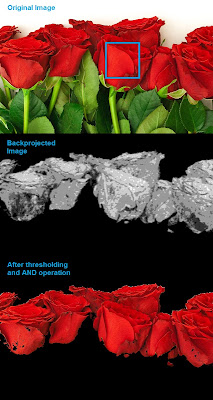Histogram Backprojection

Backprojection in OpenCV

OpenCV provides an inbuilt function cv2.calcBackProject(). Its parameters are almost same as the cv2.calcHist() function. One of its parameter is histogram which is histogram of the object and we have to find it. Also, the object histogram should be normalized before passing on to the backproject function. It returns the probability image. Then we convolve the image with a disc kernel and apply threshold. Below is my code and output :

import cv2
import numpy as np

hsv = cv2.cvtColor(img,cv2.COLOR_BGR2HSV)

hsvt = cv2.cvtColor(target,cv2.COLOR_BGR2HSV)

# calculating object histogram
roihist = cv2.calcHist([hsv],[0, 1], None, [180, 256], [0, 180, 0, 256] )

# normalize histogram and apply backprojection
cv2.normalize(roihist,roihist,0,255,cv2.NORM_MINMAX)
dst = cv2.calcBackProject([hsvt],[0,1],roihist,[0,180,0,256],1)

# Now convolute with circular disc
disc = cv2.getStructuringElement(cv2.MORPH_ELLIPSE,(5,5))
cv2.filter2D(dst,-1,disc,dst)

# threshold and binary AND
ret,thresh = cv2.threshold(dst,50,255,0)
thresh = cv2.merge((thresh,thresh,thresh))
res = cv2.bitwise_and(target,thresh)

res = np.vstack((target,thresh,res))
cv2.imwrite('res.jpg',res)


Below is the output. Here ROI is not just flower, but some green part is also included. Still output is good. On close analysis of the center image, you can see the leaf parts slightly which will be removed on threshold :Histogram Backprojection in OpenCV

Summary

So we have looked on what is Histogram backprojection, how to calculate it, how it is useful in object detection etc. It is also used in more advanced object tracking methods like camshift. We will do that later.

Regards,
Abid Rahman K.

References :

1 - "Indexing via color histograms", Swain, Michael J. , Third international conference on computer vision,1990.
2 - http://www.codeproject.com/Articles/35895/Computer-Vision-Applications-with-C-Part-II
3 - http://theiszm.wordpress.com/tag/backprojection/

## Wednesday, March 13, 2013

### Histograms - 3 : 2D Histograms

Hi friends,

In the first article, we calculated and plotted one-dimensional histogram. It is called one-dimensional because we are taking only one feature into our consideration, ie grayscale intensity value of the pixel. But in two-dimensional histograms, you consider two features. Normally it is used for finding color histograms where two features are Hue & Saturation values of every pixel.

There is a python sample in the official samples already for finding color histograms. We will try to understand how to create such a color histogram, and it will be useful in understanding further topics like Histogram Back-Projection.

2D Histogram in OpenCV

It is quite simple and calculated using the same function, cv2.calcHist(). For color histogram, we need to convert the image from BGR to HSV. (Remember, for 1D histogram, we converted from BGR to Grayscale). While calling calcHist(), parameters are :

channels = [0,1] # because we need to process both H and S plane.
bins = [180,256] # 180 for H plane and 256 for S plane
range = [0,180,0,256] # Hue value lies between 0 and 180 & Saturation lies between 0 and 256

import cv2
import numpy as np

hsv = cv2.cvtColor(img,cv2.COLOR_BGR2HSV)

hist = cv2.calcHist( [hsv], [0, 1], None, [180, 256], [0, 180, 0, 256] )


That's it.

2D Histogram in Numpy

Numpy also provides a specific function for this : np.histogram2d(). (Remember, for 1D histogram we used np.histogram() ).

import cv2
import numpy as np
from matplotlib import pyplot as plt

hsv = cv2.cvtColor(img,cv2.COLOR_BGR2HSV)

hist, xbins, ybins = np.histogram2d(h.ravel(),s.ravel(),[180,256],[[0,180],[0,256]])


First argument is H plane, second one is the S plane, third is number of bins for each and fourth is their range.

Now we can check how to plot this color histogram

Plotting 2D Histogram

Method - 1 : Using cv2.imshow()
The result we get is a two dimensional array of size 180x256. So we can show them as we do normally, using cv2.imshow() function. It will be a grayscale image and it won't give much idea what colors are there, unless you know the Hue values of different colors.

Method - 2 : Using matplotlib
We can use matplotlib.pyplot.imshow() function to plot 2D histogram with different color maps. It gives us much more better idea about the different pixel density. But this also, doesn't gives us idea what color is there on a first look, unless you know the Hue values of different colors. Still I prefer this method. It is simple and better.

NB : While using this function, remember, interpolation flag should be 'nearest' for better results.

import cv2
import numpy as np
from matplotlib import pyplot as plt

hsv = cv2.cvtColor(img,cv2.COLOR_BGR2HSV)
hist = cv2.calcHist( [hsv], [0, 1], None, [180, 256], [0, 180, 0, 256] )

plt.imshow(hist,interpolation = 'nearest')
plt.show()


Below is the input image and its color histogram plot. X axis shows S values and Y axis shows Hue.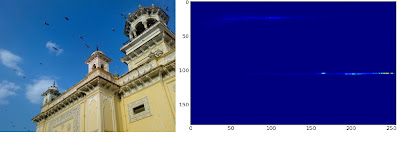2D Histogram in matplotlib with 'heat' color map

In histogram, you can see some high values near H = 100 and S = 200. It corresponds to blue of sky. Similarly another peak can be seen near H = 25 and S = 100. It corresponds to yellow of the palace. You can verify it with any image editing tools like GIMP.

Method 3 : OpenCV sample style !!
There is a sample code for color_histogram in OpenCV-Python2 samples. If you run the code, you can see the histogram shows even the corresponding color. Or simply it outputs a color coded histogram. Its result is very good (although you need to add extra bunch of lines).

In that code, the author created a color map in HSV. Then converted it into BGR. The resulting histogram image is multiplied with this color map. He also uses some preprocessing steps to remove small isolated pixels, resulting in a good histogram.

I leave it to the readers to run the code, analyze it and have your own hack arounds. Below is the output of that code for the same image as above:OpenCV-Python sample color_histogram.py output

You can clearly see in the histogram what colors are present, blue is there, yellow is there, and some white due to chessboard(it is part of that sample code) is there. Nice !!!

Summary :

So we have looked into what is 2D histogram, functions available in OpenCV and Numpy, how to plot it etc.

So this is it for today !!!

Regards,
Abid Rahman K.

## Tuesday, March 12, 2013

### Histograms - 2 : Histogram Equalization

Hi friends,

In last article, we saw what is histogram and how to plot it. This time we can learn a method for image contrast adjustment called "Histogram Equalization".

So what is it ? Consider an image whose pixel values are confined to some specific range of values only. For eg, brighter image will have all pixels confined to high values. But a good image will have pixels from all regions of the image. So you need to stretch this histogram to either ends (as given in below image, from wikipedia) and that is what Histogram Equalization does (in simple words). This normally improves the contrast of the image.Histogram Equalization

Again, I would recommend you to read the wikipedia page on Histogram Equalization for more details about it. It has a very good explanation with worked out examples, so that you would understand almost everything after reading that. And make sure you have checked the small example given in "examples" section before going on to next paragraph.

So, assuming you have checked the wiki page, I will demonstrate a simple implementation of Histogram Equalization with Numpy. After that, I will present you OpenCV function. ( If you are not interested in implementation, you can skip this and go to the end of article)

Numpy Implementation

We start with plotting histogram and its cdf (cumulative distribution function) of the image in Wikipedia page. All the functions are known to us except np.cumsum(). It is used to find the cumulative sum (cdf) of a numpy array.

import cv2
import numpy as np
from matplotlib import pyplot as plt

hist,bins = np.histogram(img.flatten(),256,[0,256])

cdf = hist.cumsum()
cdf_normalized = cdf *hist.max()/ cdf.max() # this line not necessary.

plt.plot(cdf_normalized, color = 'b')
plt.hist(img.flatten(),256,[0,256], color = 'r')
plt.xlim([0,256])
plt.legend(('cdf','histogram'), loc = 'upper left')
plt.show()Input Image and its histogram

You can see histogram lies in brighter region. We need the full spectrum. For that, we need a transformation function which maps the input pixels in brighter region to output pixels in full region. That is what histogram equalization does.

Now we find the minimum histogram value (excluding 0) and apply the histogram equalization equation as given in wiki page. But I have used here, the masked array concept array from Numpy. For masked array, all operations are performed on non-masked elements. You can read more about it from Numpy docs on masked arrays

cdf_m = np.ma.masked_equal(cdf,0)
cdf_m = (cdf_m - cdf_m.min())*255/(cdf_m.max()-cdf_m.min())
cdf = np.ma.filled(cdf_m,0).astype('uint8')


Now we have the look-up table that gives us the information on what is the output pixel value for every input pixel value. So we just apply the transform.

img2 = cdf[img]


Now we calculate its histogram and cdf as before ( you do it) and result looks like below :Histogram Equalized Image and its histogram

You can see a better contrast in the new image, and it is clear from the histogram also. Also compare the cdfs of two images. First one has a steep slope, while second one is almost a straight line showing all pixels are equi-probable.

Another important feature is that, even if the image was a darker image (instead of a brighter one we used), after equalization we will get almost the same image as we got. As a result, this is used as a "reference tool" (I don't get a more suitable than this) to make all images with same light conditions. This is useful in many cases, for eg, in face recognition, before training the face data, the images of faces are histogram equalized to make them all with same light conditions. It provides better accuracy.

OpenCV Implementation

If you are bored of everything I have written above, just leave them. You need to remember only one function to do this, cv2.calcHist(). Its input is just grayscale image and output is our image.

Below is a simple code snippet showing its usage for same image we used :

img = cv2.imread('wiki.jpg',0)
equ = cv2.equalizeHist(img)
res = np.hstack((img,equ)) #stacking images side-by-side
cv2.imwrite('res.png',res)


See the result :OpenCV Histogram Equalization

So now you can take different images with different light conditions, equalize it and check the results.

Histogram equalization is good when histogram of the image is confined to a particular region. It won't work good in places where there is large intensity variations where histogram covers a large region, ie both bright and dark pixels are present. I would like to share to SOF questions with you. Please checkout the images in the questions, analyze their histograms, check resulting images after equalization :

How can I adjust contrast in OpenCV in C?
How do I equalize contrast & brightness of images using opencv?

So I would like to wind up this article here. In this article, we learned how to implement Histogram Equalization, how to use OpenCV for that etc. So take images, equalize it and have your own hack arounds.

See you next time !!!
Abid Rahman K.

## Tuesday, March 5, 2013

### Histograms - 1 : Find, Plot, Analyze !!!

Hi,

This time, we will go through various functions in OpenCV related to histograms.

So what is histogram ? You can consider histogram as a graph or plot, which gives you an overall idea about the intensity distribution of an image. It is a plot with pixel values (ranging from 0 to 255) in X-axis and corresponding number of pixels in the image on Y-axis.

It is just another way of understanding the image. By looking at the histogram of an image, you get intuition about contrast, brightness, intensity distribution etc of that image. Almost all image processing tools today, provides features on histogram. Below is an image from "Cambridge in Color" website, and I recommend you to visit the site for more details.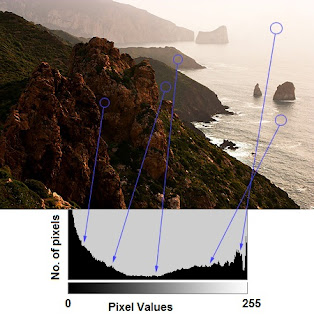Image Histogram

You can see the image and its histogram. (Remember, this histogram is drawn for grayscale image, not color image). Left region of histogram shows the amount of darker pixels in image and right region shows the amount of brighter pixels. From the histogram, you can see dark region is more than brighter region, and amount of midtones (pixel values in mid-range, say around 127) are very less.

(For more basic details on histograms, visit : http://www.cambridgeincolour.com/tutorials/histograms1.htm)

FIND HISTOGRAM

Now we have an idea on what is histogram, we can look into how to find this. OpenCV comes with an in-built function for this, cv2.calcHist(). Before using that function, we need to understand some terminologies related with histograms.

BINS :
The above histogram shows the number of pixels for every pixel value, ie from 0 to 255. ie you need 256 values to show the above histogram. But consider, what if you need not find the number of pixels for all pixel values separately, but number of pixels in a interval of pixel values? say for example, you need to find the number of pixels lying between 0 to 15, then 16 to 31, ..., 240 to 255. You will need only 16 values to represent the histogram. And that is what is shown in example given in OpenCV Tutorials on histograms.

So what you do is simply split the whole histogram to 16 sub-parts and value of each sub-part is the sum of all pixel count in it. This each sub-part is called "BIN". In first case, number of bins where 256 (one for each pixel) while in second case, it is only 16. BINS is represented by the term "histSize" in OpenCV docs.

DIMS : It is the number of parameters for which we collect the data. In our case, we collect data regarding only one thing, intensity value. So here it is 1.

RANGE : It is the range of intensity values you want to measure. Normally, it is [0,256], ie all intensity values.

So now we use cv2.calcHist() function to find the histogram. Let's familiarize with the function and its parameters :
cv2.calcHist(images, channels, mask, histSize, ranges[, hist[, accumulate]])

1 - images : it is the source image of type uint8 or float32. it should be given in square brackets, ie, "[img]".
2 - channels : it is also given in square brackets. It the index of channel for which we calculate histogram. For example, if input is grayscale image, its value is . For color image, you can pass , or  to calculate histogram of blue,green or red channel respectively.
3 - mask : mask image. To find histogram of full image, it is given as "None". But if you want to find histogram of particular region of image, you have to create a mask image for that and give it as mask. (I will show an example later.)
4 - histSize : this represents our BIN count. Need to be given in square brackets. For full scale, we pass .
5 - ranges : this is our RANGE. Normally, it is [0,256].

So let's start with a sample image. Simply load an image in grayscale mode and find its full histogram.

img = cv2.imread('home.jpg',0)
hist = cv2.calcHist([img],,None,,[0,256])


hist is a 256x1 array, each value corresponds to number of pixels in that image with its corresponding pixel value. Now we should plot it, but how ?

PLOTTING HISTOGRAM

There are two ways, 1) Short Way : use Matplotlib & 2) Long Way : use OpenCV functions

1 - Using Matplotlib:

Matplotlib comes with a histogram plotting function : matplotlib.pyplot.hist()

It directly finds the histogram and plot it. You need not use calcHist() function to find the histogram. See the code below:

import cv2
import numpy as np
from matplotlib import pyplot as plt

plt.hist(img.ravel(),256,[0,256]); plt.show()


You will get a plot as below :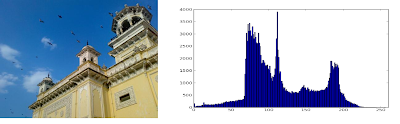Image Histogram

NOTE : Actually to find histogram, Numpy also provides you a function, np.histogram(). So instead of calcHist() function, you can try below line :

hist,bins = np.histogram(img,256,[0,256])

hist is same as we calculated before. But bins will have 257 elements, because numpy calculate bins as 0-0.99,1-1.99,2-2.99 etc. So final range would be 255-255.99. To represent that, they also add 256 at end of bins. But we don't need that 256. Upto 255 is sufficient.

Or you can use normal plot of matplotlib, which would be good for BGR plot. For that, you need to find the histogram data first. Try below code:

import cv2
import numpy as np
from matplotlib import pyplot as plt

color = ('b','g','r')
for i,col in enumerate(color):
histr = cv2.calcHist([img],[i],None,,[0,256])
plt.plot(histr,color = col)
plt.xlim([0,256])
plt.show()


You will get a image as below :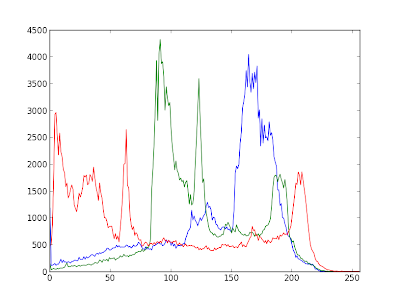Histogram showing different channels

You can deduct from the above graph that, blue has some high value areas(obviously it should be the due to sky)

2 - Using OpenCV functions :

Well, here you adjust the values of histograms along with its bin values to look like x,y coordinates so that you can draw it using cv2.line() or cv2.polyline() function to generate same image as above. This is already available with OpenCV-Python2 official samples. You can check that : https://github.com/Itseez/opencv/blob/master/samples/python2/hist.py . I had already mentioned it in one of my very early articles : Drawing Histogram in OpenCV-Python

Now we used calcHist to find the histogram of full image. What if you want to find some regions of an image? Just create a mask image with white color on the region you want to find histogram and black otherwise. I have demonstrated it while answering a SOF question. So I would like you to read that answer (http://stackoverflow.com/a/11163952/1134940). Just for a demo, I provide the same images here :Application of Mask
Due to resizing, histogram plot clarity is reduced. But I hope you can write your own code and analyze it.

SUMMARY
In short, we have seen what is image histogram, how to find and interpret histograms, how to plot histograms etc. It is sufficient for today. We will look into other histogram functions in coming articles.

Hope you enjoyed it !!! Feel free to share !!!

Abid Rahman K.

## Sunday, January 27, 2013

### K-Means Clustering - 3 : Working with OpenCV

Hi,

In the previous articles, K-Means Clustering - 1 : Basic Understanding and K-Means Clustering - 2 : Working with Scipy, we have seen what is K-Means and how to use it to cluster the data. In this article, We will see how we can use K-Means function in OpenCV for K-Means clustering.

OpenCV documentation for K-Means clustering : cv2.KMeans()

Function parameters :

Input parameters :

1 - samples : It should be of np.float32 data type, and as said in previous article, each feature should be put in a single column.

2 - nclusters(K
) : Number of clusters

3 - criteria : It is the algorithm termination criteria. Actually, it should be a tuple of 3 parameters. They are ( type, max_iter, epsilon ):
3.a - type of termination criteria : It has 3 flags as below:
- cv2.TERM_CRITERIA_EPS - stop the algorithm iteration if specified accuracy, epsilon, is reached.
- cv2.TERM_CRITERIA_MAX_ITER - stop the algorithm after the specified number of iterations, max_iter.
- cv2.TERM_CRITERIA_EPS + cv2.TERM_CRITERIA_MAX_ITER - stop the iteration when any of the above condition is met.

3.b - max_iter - An integer specifying maximum number of iterations.
3.c - epsilon - Required accuracy

4 - attempts : Flag to specify the number of times the algorithm is executed using different initial labellings. The algorithm returns the labels that yield the best compactness. This compactness is returned as output.

5 - flags : This flag is used to specify how initial centers are taken. Normally two flags are used for this : cv2.KMEANS_PP_CENTERS and cv2.KMEANS_RANDOM_CENTERS. (I didn't find any difference in their results, so I don't know where they are suitable. For time-being, I use second one in my examples).

Output parameters:

1 - compactness : It is the sum of squared distance from each point to their corresponding centers.

2 - labels : This is the label array (same as 'code' in previous article) where each element marked '0', '1'.....

3 - centers : This is array of centers of clusters.

Now let's do the same examples we did in last article. Remember, we used random number generator to generate data, so data may be different this time.

1 - Data with Only One Feature:

Below is the code, I have commented on important parts.

import numpy as np
import cv2
from matplotlib import pyplot as plt

x = np.random.randint(25,100,25)
y = np.random.randint(175,255,25)
z = np.hstack((x,y))
z = z.reshape((50,1))

# data should be np.float32 type
z = np.float32(z)

# Define criteria = ( type, max_iter = 10 , epsilon = 1.0 )
criteria = (cv2.TERM_CRITERIA_EPS + cv2.TERM_CRITERIA_MAX_ITER, 10, 1.0)

# Apply KMeans
ret,labels,centers = cv2.kmeans(z,2,criteria,10,cv2.KMEANS_RANDOM_CENTERS)

# Now split the data depending on their labels
A = z[labels==0]
B = z[labels==1]

# Now plot 'A' in red, 'B' in blue, 'centers' in yellow
plt.hist(A,256,[0,256],color = 'r')
plt.hist(B,256,[0,256],color = 'b')
plt.hist(centers,32,[0,256],color = 'y')
plt.show()


Below is the output we get :KMeans() with one feature set

2 - Data with more than one feature :

Directly moving to the code:

import numpy as np
import cv2
from matplotlib import pyplot as plt

X = np.random.randint(25,50,(25,2))
Y = np.random.randint(60,85,(25,2))
Z = np.vstack((X,Y))

# convert to np.float32
Z = np.float32(Z)

# define criteria and apply kmeans()
criteria = (cv2.TERM_CRITERIA_EPS + cv2.TERM_CRITERIA_MAX_ITER, 10, 1.0)
ret,label,center = cv2.kmeans(Z,2,criteria,10,cv2.KMEANS_RANDOM_CENTERS)

# Now separate the data, Note the flatten()
A = Z[label.flatten()==0]
B = Z[label.flatten()==1]

# Plot the data
plt.scatter(A[:,0],A[:,1])
plt.scatter(B[:,0],B[:,1],c = 'r')
plt.scatter(center[:,0],center[:,1],s = 80,c = 'y', marker = 's')
plt.xlabel('Height'),plt.ylabel('Weight')
plt.show()


Note that, while separating data to A and B, we used label.flatten(). It is because 'label' returned by the OpenCV is a column vector. Actually, we needed a plain array. In Scipy, we get 'label' as plain array, so we don't need the flatten() there in Scipy. To understand more, check the 'label' in both the cases.

Below is the output we get :KMeans() with two feature sets

3 - Color Quantization :

import numpy as np
import cv2
from matplotlib import pyplot as plt

Z = img.reshape((-1,3))

# convert to np.float32
Z = np.float32(Z)

# define criteria, number of clusters(K) and apply kmeans()
criteria = (cv2.TERM_CRITERIA_EPS + cv2.TERM_CRITERIA_MAX_ITER, 10, 1.0)
K = 8
ret,label,center = cv2.kmeans(Z,K,criteria,10,cv2.KMEANS_RANDOM_CENTERS)

# Now convert back into uint8, and make original image
center = np.uint8(center)
res = center[label.flatten()]
res2 = res.reshape((img.shape))

cv2.imshow('res2',res2)
cv2.waitKey(0)
cv2.destroyAllWindows()


Below is the output we get :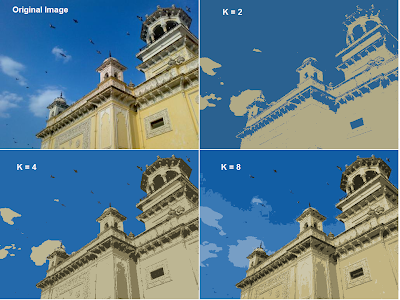Color Quantization with KMeans Clustering

Summary :

So finally, We have seen how to use KMeans clustering with OpenCV. I know, I haven't explained much in this article, because it is same as the previous article. Just a function is changed.

So this series on KMeans Clustering algorithm ends here.

Feel free to share it with your friends....

Regards,
Abid Rahman K.

## Friday, January 11, 2013

### Contours - 5 : Hierarchy

Hi,

In the last few articles on contours, you have worked with several functions related to contours provided by OpenCV. But when we found the contours in image using cv2.findContours() function, we have passed two arguments additional to source image. They are Contour Retrieval Mode and Contour Approximation Method. We usually passed cv2.RETR_LIST or cv2.RETR_TREE for first argument and cv2.CHAIN_APPROXIMATE_SIMPLE for second argument, and they worked nice. But what do they actually mean ?

Also, in the output, we got two arrays, one is our contours, and one more output which we named as 'hierarchy' (Please checkout the codes in previous articles). But we never used this hierarchy anywhere. Then what is this hierarchy and what is it for ? What is its relationship with the previous mentioned function arguments ?

I don't know how important is this topic. Mainly because, I have never worried about hierarchy and other arguments in any of my projects. And there was no reason to. But I am sure, there might be some people who benefit from these features, otherwise OpenCV devs wouldn't have spent time to introduce such a feature. So whatever may be its use, let's just go through it. :)

So, what is this "hierarchy" ?

Normally we use the findContours() function to detect objects in an image, right ? Sometimes objects are in different locations. But in some cases, some shapes are inside other shapes. Just like nested figures. In this case, we call outer one as parent and inner one as child. This way, contours in an image has some relationship to each other. And we can specify how one contour is connected to each other, like, is it child of some other contour, or is it a parent etc. Representation of this relationship is called the hierarchy.

Consider an example image below :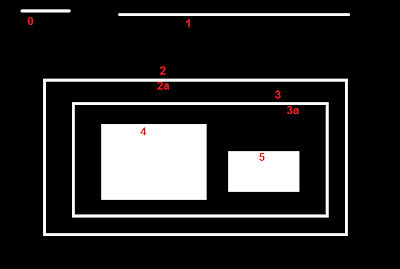Hierarchy Representation

In this image, there are a few shapes which I have numbered from 0 to 5. 2 and 2a denotes the external and internal contour of the outermost box..

Here, contours 0,1,2 are external or outermost. We can say, they are in hierarchy-0 or simply they are in same hierarchy level.

Next comes contour 2a. It can be considered as a child of contour 2 (or in opposite way, contour 2 is parent of contour 2a). So let it be in hierarchy-1. Similarly contour 3 is child of contour 2 and it comes in next hierarchy. Finally contours 4,5 are the children of 3a, and they come in the last hierarchy level. From the way I numbered the boxes, I would say contour 4 is the first child of contour 3a.

I mentioned these things to understand terms like "same hierarchy level", "external contour", "child contour", "parent contour", "first child" etc. Now let's get into OpenCV.

Hierarchy Representation in OpenCV :

So each contour has its own information regarding what hierarchy it is, who is its child, who is its parent etc. OpenCV represents it as an array of four values : [Next, Previous, First_Child, Parent]
"Next denotes next contour at the same hierarchical level."
For eg, take contour 0 in our picture. Who is next contour in its same level ? It is contour 1. So simply put it as 1. Similarly for Contour 1, next is contour 2. So Next = 2.

What about contour 2? There is no next contour in same level. So simply, put it as -1.

What about contour 4? It is in same level with contour 5. So its next contour is contour 5.
"Previous denotes previous contour at the same hierarchical level."
It is same as above. Previous contour of contour 1 is contour 0 in same level. Similarly for contour 2, it is contour 1. And for contour 0, there is no previous, so put it as -1.
"First_Child denotes its first child contour."
I think there is no need of any explanation. For contour 2, child is contour 2a. So it gets the corresponding index value of contour 2a.

What about contour 3a? It has two children. But we take only first child. And it is contour 4. So First_Child = 4 for contour 3a.
"Parent denotes index of its parent contour"
It is just opposite of First_Child. Both for contour 4 and 5, parent contour is contour 3a. For 3a, it is contour 3 and so on.

If there is no child or parent, that field is taken as -1.

So now we know about the hierarchy style used in OpenCV, we can check into Contour Retrieval Modes in OpenCV with the help of same image given above. ie what do flags like cv2.RETR_LIST, cv2.RETR_TREE, cv2.CCOMP, cv2.EXTERNAL etc mean?

Contour Retrieval Mode :

This is the second argument in cv2.findContours() function. Lets' understand each flag one-by-one.

cv2.RETR_LIST :

This is the simplest of the four flags (from explanation point of view). It simply retrieves all the contours, but doesn't create any parent-child relationship. "Parents are kids are equal under this rule, and they are just contours". ie they all belongs to same hierarchy level.

So here, 3rd and 4th term in hierarchy array is always -1. But obviously, Next and Previous terms will have their corresponding values. Just check it yourself and verify it.

Below is the result I got, and each row is hierarchy details of corresponding contour. For eg, first row corresponds to contour 0. Next contour is contour 1. So Next = 1. There is no previous contour, so Previous = 0. And the remaining two, as told before, it is -1.

>>> hierarchy
array([[[ 1, -1, -1, -1],
[ 2,  0, -1, -1],
[ 3,  1, -1, -1],
[ 4,  2, -1, -1],
[ 5,  3, -1, -1],
[ 6,  4, -1, -1],
[ 7,  5, -1, -1],
[-1,  6, -1, -1]]])


This is the good choice to use in your code, if you are not using any hierarchy features.

cv2.RETR_EXTERNAL

If you use this flag, it returns only extreme outer flags. All child contours are left behind. "We can say, under this law, Only the eldest in every family is taken care of. It doesn't care about other members of the family :)".

So, in our image, how many extreme outer contours are there? ie at hierarchy-0 level?. Only 3, ie contours 0,1,2, right? Now try to find the contours using this flag. Here also, values given to each element is same as above. Compare it with above result. Below is what I got :
>>> hierarchy
array([[[ 1, -1, -1, -1],
[ 2,  0, -1, -1],
[-1,  1, -1, -1]]])


You can use this flag if you want to extract only the outer contours. It might be useful in some cases.

cv2.RETR_CCOMP :

This flag retrieves all the contours and arranges them to a 2-level hierarchy. ie external contours of the object (ie its boundary) are placed in hierarchy-1. And the contours of holes inside object (if any) is placed in hierarchy-2. If any object inside it, its contour is placed again in hierarchy-1 only. And its hole in hierarchy-2 and so on.

Just consider the image of a "big white zero" on a black background. Outer circle of zero belongs to first hierarchy, and inner circle of zero belongs to second hierarchy.

We can explain it with a simple image. Here I have labelled the order of contours in red color and the hierarchy they belongs to, in green color (either 1 or 2). The order is same as the order OpenCV detects contours.

So consider first contour, ie contour-0. It is hierarchy-1. It has two holes, contours 1&2, and they belong to hierarchy-2. So for contour-0, Next contour in same hierarchy level is contour-3. And there is no previous one. And its first is child is contour-1 in hierarchy-2. It has no parent, because it is in hierarchy-1. So its hierarchy array is [3,-1,1,-1]

Now take contour-1. It is in hierarchy-2. Next one in same hierarchy (under the parenthood of contour-1) is contour-2. No previous one. No child, but parent is contour-0. So array is [2,-1,-1,0].

Similarly contour-2 : It is in hierarchy-2. There is not next contour in same hierarchy under contour-0. So no Next. Previous is contour-1. No child, parent is contour-0. So array is [-1,1,-1,0].

Contour - 3 : Next in hierarchy-1 is contour-5. Previous is contour-0. Child is contour-4 and no parent. So array is [5,0,4,-1].

Contour - 4 : It is in hierarchy 2 under contour-3 and it has no sibling. So no next, no previous, no child, parent is contour-3. So array is [-1,-1,-1,3].

Remaining you can fill up. This is the final answer I got:

>>> hierarchy
array([[[ 3, -1,  1, -1],
[ 2, -1, -1,  0],
[-1,  1, -1,  0],
[ 5,  0,  4, -1],
[-1, -1, -1,  3],
[ 7,  3,  6, -1],
[-1, -1, -1,  5],
[ 8,  5, -1, -1],
[-1,  7, -1, -1]]])


So where do we can apply this ? I don't have any good application now. One application would be in OCR. Those who have checked my article "Simple Digit Recognition OCR in OpenCV-Python" would have noticed that I used area as a constraint to remove the contours of holes inside numbers like 8,9,0,6 etc. I found that area by checking a lot of values. Instead, I should have used this feature to filter out holes inside the numbers.(To be honest, I had no idea regarding the hierarchy when I wrote that code.)

UPDATE : You can find a simple demo of practical application of cv2.RETR_CCOMP in this SOF link : http://stackoverflow.com/a/14279746/1134940

cv2.RETR_TREE :

And this is the final guy, Mr.Perfect. It retrieves all the contours and creates a full family hierarchy list. "It even tells, who is the grandpa, father, son, grandson and even beyond... ".

For examle, I take above image, rewrite the code for cv2.RETR_TREE, reorder the contours as per the result given by OpenCV and analyze it. Again, red letters give the contour number and green letters give the hierarchy order.

Take contour-0 : It is in hierarchy-0. Next contour in same hierarchy is contour-7. No previous contours. Child is contour-1. And no parent. So array is [7,-1,1,-1].

Take contour-2 : It is in hierarchy-1. No contour in same level. No previous one. Child is contour-2. Parent is contour-0. So array is [-1,-1,2,0].

And remaining, try yourself. Below is the full answer:

>>> hierarchy
array([[[ 7, -1,  1, -1],
[-1, -1,  2,  0],
[-1, -1,  3,  1],
[-1, -1,  4,  2],
[-1, -1,  5,  3],
[ 6, -1, -1,  4],
[-1,  5, -1,  4],
[ 8,  0, -1, -1],
[-1,  7, -1, -1]]])


I am not sure where you can use it.

So this is what Contour Retrieval Mode.

Next we will deal with third argument in cv2.findContours(), ie Contour Approximation method.

Contour Approximation Method

There are 3 flags under this category, but I am discussing only the first two - cv2.CHAIN_APPROX_NONE and cv2.CHAIN_APPROX_SIMPLE.

First one finds all the points on the contour or the boundary. But actually do we need all the points? For eg, you found the contour of a straight line. Do you need all the points on the line to represent that line? No, we need just two end points of that line. This is what second flag does. It removes all redundant points and compresses the contour.

It can be easily visualized as follows. Take an image with upright rectangle in it. Find the contours using both the flags (Take second argument as cv2.RETR_LIST). First compare number of points in each contour. Now plot each point in both the contour on the rectangle and compare the result. See it below :contours using cv2.CHAIN_APPROX_SIMPLE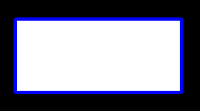contours using cv2.CHAIN_APPROX_NONE

In first case, you can see a blue boundary. It is because, all the points plotted are touching each other. Actually they are distinct points. And it has 734 points in the array. But second method has only four points in four corners. That is really a good difference. Second method is a good improvement, both in memory consumption and performance.

**********************************************************************************

So I think you might have got a simple intuitive understanding regarding concept of hierarchy in OpenCV. As I mentioned in the beginning of this article, I don't know how important is this topic, since I have never used this. If I find any application using this hierarchy, I will provide the links here.